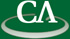## Algorithms for Symbolic Polynomials

### 9:30am, Tuesday November 25th, 2014, Room K9509.

```Abstract

This talk is about polynomials where the exponents are not known
in advance, such as \$x^{2n} - 1\$.  There are various operations we
want to be able to do, such as squaring the value to get \$x^{4n}-2x^{2n}+1\$,
or differentiating it to get \$2nx^{2n-1}\$.  Expressions of this sort
arise frequently in practice, for example in the analysis of algorithms,
and it is difficult to work with them effectively in current computer
algebra systems.

We consider the case where multivariate polynomials can have exponents
which are themselves integer-valued multivariate polynomials, and we present
algorithms to compute their GCD, factorization and functional decomposition.

The algorithms fall into two families: algebraic extension methods and
interpolation methods.  The first family of algorithms uses the algebraic
independence of  \$x\$, \$x^n\$, \$x^{n^2}\$, \$x^{nm}\$, etc, to solve related
problems with more indeterminates.  Some subtlety is needed to handle
fixed divisors of the exponent polynomials.  The second family of
algorithms uses evaluation and interpolation of the exponent polynomials.
While these methods can run into unlucky evaluation points, in many cases
they can be more appealing.

```